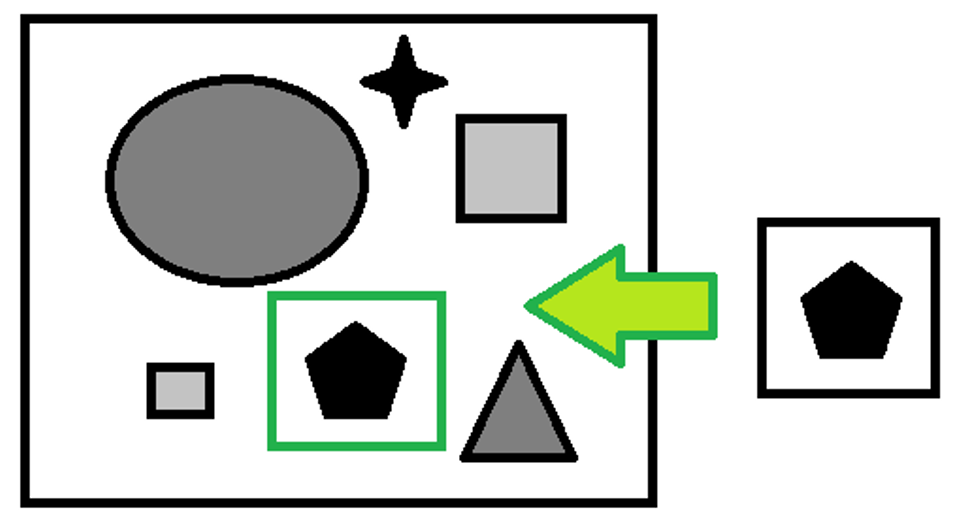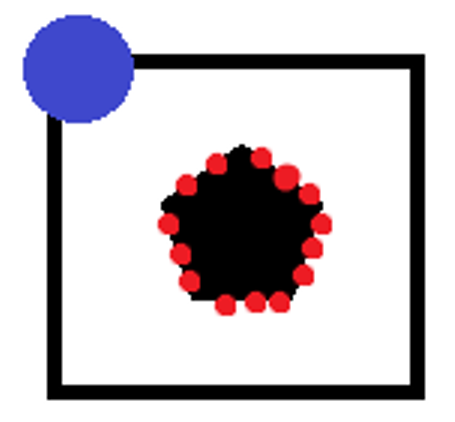## Template matching

Template matching is a technique for recognizing which parts of an image match a template that represents a model image.

Clearly the template is smaller than the image to be analyzed.There are many techniques to do this, the main ones being:

• Comparing the pixel values of the template and image.
• One example is SAD (sum of absolute difference) which associates a new size matrix to the image, equivalent to:

- Number of rows = number of image rows - number of template rows

- Number of columns = number of image columns - number of template columns

The values of each element of the matrix will be:

M(r,c)=sum_(r',c')abs(T(r',c')-I(r+r',c+c'))

Where r and c stand for the row and column coordinates and the sum is constructed on r’,c’ the template coordinates, therefore between 0 and the value of the template rows/columns. The closer this value is to zero the more likely the analysed portion will match the template. This approach is greatly affected by the absolute value of the pixel. The soundness of the search can be increased with a simple normalization based on the averages of the pixel values of the template and of the image.

• Comparison between template features and image features. One example is shape matching which compares the vectors of the gradients of the image outlines.Some points of the outline (red dots) are extracted from the template, the positions of these points are saved with respect to a coordinate of reference (blue dot) which in our case has coordinate (0,0), the vectors of the gradients for each point of the template in question are saved.

The vectors of the gradients of the template and of the image are then compared, scrolling the coordinates of the reference point along the entire image. A matrix is associated with dimensionality equal to:

- Number of rows = number of image rows - number of template rows

- Number of columns = number of image columns - number of template columns

With values

M(r,c)=1/nsum_(i=1)^n((:GI_i|GT_i:))/(abs(GI_i)abs(GT_i))

With the sum constructed on the subset of the selected template points. Therefore, the gradient vector of point i of the image GI with coordinate (u,v)=(r,c)+(xi,yi) – where (r,c) is the new offset and (xi,yi) the relative position of the point to be analyzed with respect to the reference point in the template – is compared with the gradient of the point with coordinates (xi,yi) of the template GT.

Thanks to normalization, these values are always between -1 and 1. If the orientation of the gradient is irrelevant, but one is only concerned in its direction, the formula can be modified:

M(r,c)=1/nsum_(i=1)^nabs((:GI_i|GT_i:))/(abs(GI_i)abs(GT_i))

In this case, the values will still be between 0 and 1.

The closer the value is to 1, the more likely it is for the image to contain the required template.

The methods put forth above have the scale and rotation unchanged, but they can be modified to be adapted to this purpose.

Next →